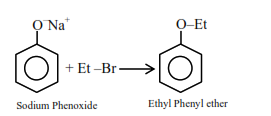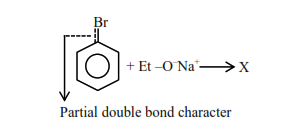# Given below are two statements : one is labelled as`
Question:

Given below are two statements : one is labelled as

Assertion (A) and the other is labelled as Reason (R).

Assertion (A): Synthesis of ethyl phenyl ether may be achieved by Williamson synthesis.

Reason (R): Reaction of bromobenzene with sodium ethoxide yields ethyl phenyl ether. In the light of the above statements, choose the most appropriate answer from the options given below:

1. Both (A) and (R) are correct and (R) is the correct explanation of (A)

2. (A) is correct but (R) is not correct

3.  (A) is not correct but (R) is correct

4.  Both (A) and (R) are correct but (R) is NOT the correct explanation of (A)

Correct Option: , 2

Solution: